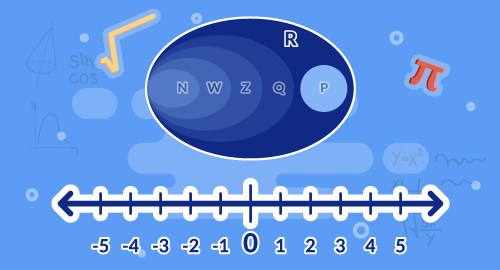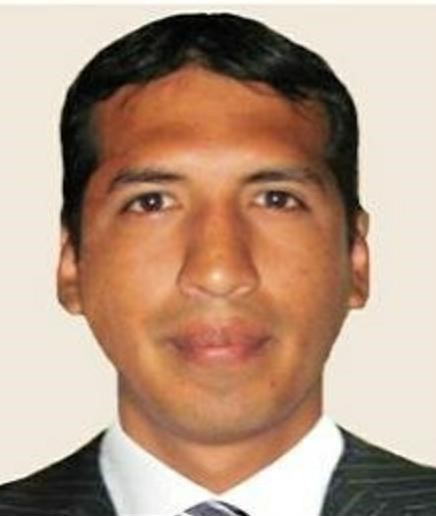#### Need Help?

Get in touch with usSAT/ACT

# Real Numbers

Maths

Every rational number can be converted as decimal expansion. Use rational approximation of irrational number. Use square root and cube root to represent solution to the equations. Evaluate square root and cube root for perfect squares and perfects cubes respectively.

##### Course Duration
•1 Weeks5 Sessions
•50 mins per session
##### Tutor## What you'll learn

• 1

Convert rational numbers to decimals.

• 2

Identify the number is rational/ irrational.

• 3

Find square root and cube root of a rational number.

• 4

Solve equations involving square root and cube root.

## Skills you'll learnProblem solving